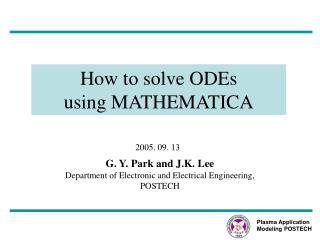DownloadDownload PresentationHow to solve ODEs using MATHEMATICA

# How to solve ODEs using MATHEMATICA

Download Presentation## How to solve ODEs using MATHEMATICA

- - - - - - - - - - - - - - - - - - - - - - - - - - - E N D - - - - - - - - - - - - - - - - - - - - - - - - - - -
##### Presentation Transcript

1. Plasma Application Modeling POSTECH How to solve ODEs using MATHEMATICA 2005. 09. 13 G. Y. Park and J.K. Lee Department of Electronic and Electrical Engineering, POSTECH

2. Plasma Application Modeling POSTECH Contents • Basic usages of MATHEMATICA • Solving ODEs • - Euler’s method • - Predictor-Corrector method ( second-order ) • - Forth-order Runge-Kutta method

3. Plasma Application Modeling POSTECH References • Textbook • - ‘Numerical and Analytical Methods for Scientists and Engineers Using Mathematica’, Daniel Dubin, Wiley, 2003 • Web Lecture • : http://www.mathought.com/main.htm

4. Plasma Application Modeling POSTECH Notebook : working window ( *.nb ) Standard Screen Palettes ( File – Palettes - ) : a collection of numerical expressions or characters

5. Plasma Application Modeling POSTECH Basic Usages (1) • Shift + enter : Execution (shift + enter) (shift + enter) • In indicates input contents in the line. • Out indicates the result of In • % : a symbol indicating the result obtained right before.

6. Plasma Application Modeling POSTECH • Font size • : Format - Size - Basic Usages (2) • application of Palettes

7. Plasma Application Modeling POSTECH Basic Usages (3) • The names of most of functions included start with a capital letter. • included constants • - π→ Pi • - ∞ → Infinite • - e → E • - i =→ I • ? (function) or ?? (function) • : shows the usage of function or options

8. Plasma Application Modeling POSTECH Basic Usages (4) • symbol calculation • The symbol “ * ” for a product can be replaced by “ blank”.

9. Plasma Application Modeling POSTECH Common feature Different feature Basic Usages (3) • == ( equality ), = ( substitution ), := ( definition ) < g[x] = eq. & g[x] := eq. >

10. Plasma Application Modeling POSTECH Common feature Different feature Basic Usages (3) < g[x_] = eq. & f[x_] := eq. >

11. Plasma Application Modeling POSTECH Basic Usages (4) • Plot[ function, {variable, a, b}, options] : drawing 2-D graph between a and b

12. Plasma Application Modeling POSTECH Basic Usages (3) • PlotStyle : a option for coloring • Input - Color Selector

13. Basic Usages (3) • PlotLabel : a option for labeling at top of graph • AxesLabel : a option for labeling at axes • AspectRatio : a option for adjusting the ratio of vertical to horizontal • PlotRange : a option for resticting range to plot

14. Plasma Application Modeling POSTECH Basic Usages (3) • DisplayFunction → Identity : a option for not showing a graph, just memorizing it. • Show : a function to display graphs which have been shown or memorized • DisplayFunction -> \$DisplayFunction : a option for showing a graph memorized.

15. Plasma Application Modeling POSTECH • Since path is assigned up to StandardPackages, just sub-paths • should be written. • The symbol ` is used in Mathematica instead of \. Basic Usages (3) • Package

16. Plasma Application Modeling POSTECH Basic Usages (3) • Table [contents, {range of loop}]

17. Plasma Application Modeling POSTECH • Since Do, For, While don’t show results and save results, functions such as Print[] should be used for checking results. • That’s a difference among Table and above functions. Basic Usages (3) • Do [exp, {i,min,max,d}] • For [start, test, i++, body] • While [test, body]

18. Plasma Application Modeling POSTECH Basic Usages (3) • Module [ {local variables}, contents ] • : The variables written at {} in Module[ ] are used as local variables which doesn’t affect global variables with same characters and can’t be used out of Module[ ].

19. Plasma Application Modeling POSTECH Solving ODEs - Euler’s method - Predictor-Corrector method ( second-order ) - Forth-order Runge-Kutta method

20. Plasma Application Modeling POSTECH Euler’s method (1)

21. Plasma Application Modeling POSTECH Euler’s method (2)

22. Predictor-Corrector method

23. Plasma Application Modeling POSTECH Forth-order Runge-Kutta method ( based on the Simpson’s 1/3 rule )

24. Example 9.1 ( Nakamura )